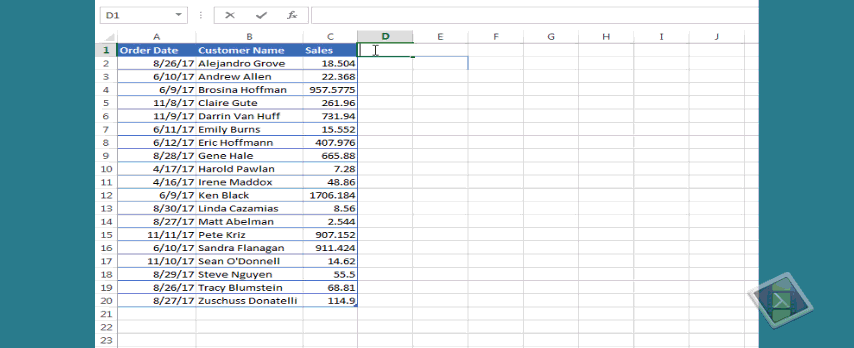#### Email Us

Follows

Every organizationÂ requires weekly summaries to monitor peak and off-peak periods. This is mostly so when you are in the production section.

Excel has a beautiful but overlooked function (WEEKNUM) that returns an integer representing the week in the year (1 to 53).

For anyone not familiar with WEEKNUM function, it contains 2 arguments; Serial_number ( the date to return the week number for) and an optional Return_type (an integer that defines when the week starts).

NB: If the return type is omitted, function defaults to week start on Sunday all the way to Saturday

`=WEEKNUM(Â serial_number,Â [return_type]Â )`
[return_type] Meaning
1 Week from Sunday to Saturday
2 Week from Monday to Sunday
11 WeekÂ  from Monday to Sunday
12 Week from Tuesday to Monday
13 Week from Wednesday to Tuesday
14 WeekÂ  from Thursday to Wednesday
15 WeekÂ  from Friday to Thursday
16 WeekÂ  from Saturday to Friday
17 Week from Sunday to Monday
21 WeekÂ  from Monday to Sunday

Beautiful as the function is, it has one major weakness, WEEKNUMÂ  doesn’t accept a range argument e.g. `=WEEKNUM(K11:K110)`Â just returns #VALUE! error.

So, How do you get week numbers in an array given a range of dates?

Do you need to always use a helper column to convert dates to week numbers before any analysis is done?

1. SUMIF
2. SUMPRODUCT
3. SUM & IF
4. PIVOT TABLES

## SUM BY WEEK USING SUMIF

Given the below data, Show totals per week.Since WEEKNUM does not accept a range argument, then we have to create a helper column so that we can be able to use SUMIF## SUM BY WEEK USING SUMPRODUCT

It is not entirely true that WEEKNUMÂ does not accept a range argument. It can beÂ forced to accept range by adding zero to a range i.e.

`=WEEKNUM(A1:A25+0)`

The above function does not return an error but an array of week numbers from the given dates range.

Since SUMPRODUCT function comfortably handles arrays, we can use this array of week numbers to create a summary as shown below.```=SUMPRODUCT(
(WEEKNUM(Table13[Order Date]+0)=[@[Week No.]])
*Table13[Sales]
)```

How it works:

â–ºWEEKNUM(Table13[Order Date]+0) returns an array of week numbers for the given date range

`{34;23;23;45;45;24;24;35;16;16;23;35;35;45;23;45;35;34;35}`

â–ºWEEKNUM(Table13[Order Date]+0)=[@[Week No.]] returns an array of TRUE/FALSE based on the week number criteria.

```{TRUE;FALSE;FALSE;FALSE;FALSE;FALSE;FALSE;FALSE;FALSE;
FALSE;FALSE;FALSE;FALSE;FALSE;FALSE;FALSE;FALSE;TRUE;FALSE}```

â–º(WEEKNUM(Table13[Order Date]+0)=[@[Week No.]])*Table13[Sales]Â  During this multiplication, the above array of TRUE/FALSE is converted into its numeric equivalent of 1/0Â  which is multiplied with the sales figure. Below is the result;

```{18.504;0;0;0;0;0;0;0;0;0;0;0;0;0;0;0;0;68.81;0}

--this array is fed to SUMPRODUCT for the final additions```
`=SUMPRODUCT({18.504;0;0;0;0;0;0;0;0;0;0;0;0;0;0;0;0;68.81;0})=87.314`

## SUM BY WEEK USING SUM & IF FUNCTION

This is an array function that uses the same principles as SUMPRODUCT

`{=SUM(IF(WEEKNUM(Table135[Order Date]+0)=[@[Week No.]],Table135[Sales]))}`

How it works:

â–ºWEEKNUM(Table13[Order Date]+0)=[@[Week No.]] returns an array of TRUE/FALSE based on the week number criteria.

```{TRUE;FALSE;FALSE;FALSE;FALSE;FALSE;FALSE;FALSE;FALSE;FALSE;
FALSE;FALSE;FALSE;FALSE;FALSE;FALSE;FALSE;TRUE;FALSE}```

â–ºIF function returns only the Sales values if the test is TRUE, otherwise returns FALSE

```{18.504;FALSE;FALSE;FALSE;FALSE;FALSE;FALSE;
FALSE;FALSE;FALSE;FALSE;FALSE;FALSE;FALSE;FALSE;FALSE;
FALSE;68.81;FALSE}```

â–ºSince SUM function ignores texts, It just sums up the numbers

```=SUM({18.504;FALSE;FALSE;FALSE;FALSE;FALSE;FALSE;FALSE;FALSE;
FALSE;FALSE;FALSE;FALSE;FALSE;FALSE;FALSE;FALSE;68.81;FALSE})=87.314```

## SUM BY WEEK USING PIVOT TABLES

This is the simplest and easiest method.

Its only major drawback is that it will give you the week’s date range but not the week number.

NB: The trick in using the pivot table is knowing how to group the dates into weeks. Watch the video again.

## Conclusion

There is now no need to lose your hair over worrying about how to do weekly summaries.

You can use the same techniques taught above to do Average and Count.

If I have missed something, share.

### RELATED ARTICLES

2 WAY LOOKUP IN EXCEL